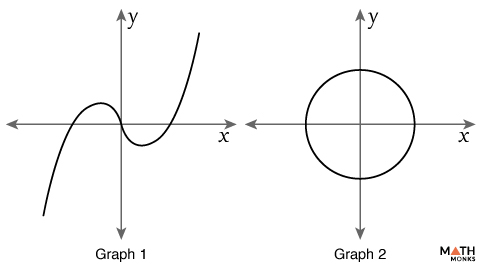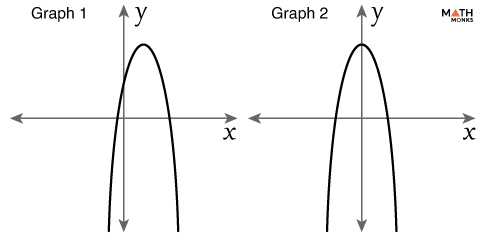# Graph Symmetry

A graph is said to have symmetry if it can divided into two equal parts along a line such that one part is an exact mirror image of the other. The concept is useful to graph an equation.

## Types

Graph symmetry is of 3 types:

### x-axis Symmetry

A graph is symmetric about the x-axis when the points (x, y) and (x, -y) are present on the same graph. A graph will have x-axis symmetry if we get an equivalent equation to the original one when we replace y with –y.

Let us test the symmetry of a graph with the equation 6 + xy4 = 0

x-axis symmetry test: replacing y with –y:

6 + x(-y)4 = 0

⇒ 6 + xy4 = 0 (this is the original equation)

Thus the graph is symmetric about the x-axis.

### y-axis Symmetry

A graph is symmetric about the y-axis when the points (x, y) and (-x, y) are present on the same graph. A graph will have y-axis symmetry if we get an original equation to the original one when we replace x with –x.

Let us test the symmetry of a graph with the equation y = x4 +3x2

x-axis symmetry test: replacing y with –y:

-y = x4 +3x2

Y = – (x4 +3x2), this is not the equivalent equation.

y-axis symmetry test: replacing x with –x.

So, y = (-x)4 +3(-x)2

y = x4 +3x2 (this is the original equation)

Thus the graph is symmetric about the y-axis.

### Origin Symmetry

A graph is symmetric about the origin when the points (x, y) and (-x,-y) are present on the same graph. A graph will have symmetry about the origin if we get an equivalent equation to the original one when we replace y with –y and also x with –x.

Let us test the symmetry of a graph with the equation xy = 4

x-axis symmetry test: replacing y with –y:

x(-y) = 4

-xy = 4, (this is not the original equation)

y-axis symmetry test: replacing x with –x.

(-x)y = 4

-xy = 4, (this is also not the original equation)

Origin symmetry test: replacing x with –x and y with -y.

(-x)(-y) = 4

xy = 4, (this is the original equation)

So, the graph is symmetric about the origin.

## How to Find the Axis of Symmetry on a Graph

For a parabola the axis of symmetry always passes through the vertex. The x -coordinate of the vertex is the equation of the axis of symmetry of the parabola.

For a quadratic function in standard form y = ax2 + bx + c, the axis of symmetry is x = -b/2a

Now, let us find the axis of symmetry of the given quadratic graph.

The given graph shows line symmetry about the y-axis.

So for a graph y = x2 – 4

Axis of symmetry is x = -b/2a, here a = 1, b = 0

∴ x = 0

## Solved Examples

Prove the symmetry of the graph y = x2

Solution:

x-axis symmetry test: Replacing y with –y,
-y = x2 (this is not equivalent to the original equation)
y-axis symmetry test: Replacing x with –x,
y = (-x) 2
= x2
∴ The graph is symmetric about the y-axis

Test the symmetry of the graph y = x3

Solution:

x-axis symmetry test: Replacing y with –y,
-y = x3 (this is not equivalent to the original equation)
(this is not equivalent to the original equation)
y = (-x)3
= -x3 (this is not equivalent to the original equation)
Origin symmetry test: replacing x with –x and y with -y.
-y = (-x)3
-y = -x3
Y = x3, (this is the original equation)
∴ The graph is symmetric about the origin.Which graph shows rotational symmetry and which one shows line symmetry in the figure alongside?

Solution:

Graph 2 shows rotational symmetry since the graph is a perfect circle about the origin.
Graph 1 shows point symmetry where the central point is the origin.Which graph shows line symmetry about the y-axis in the figure alongside?

Solution:

Graph 2 shows line symmetry about the y-axis.
Graph 1 does not have the y-axis as its line of symmetry.